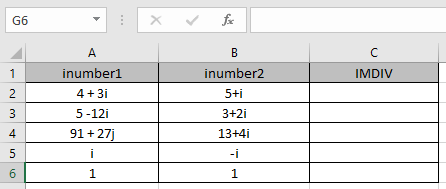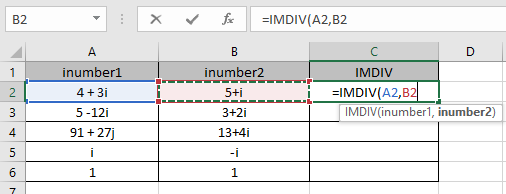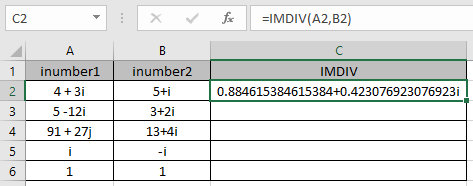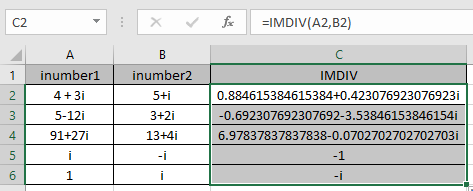# How to use the IMDIV Function in Excel

COMPLEX number (inumber) in excel derived for mathematical number having real and imaginary coefficients. In mathematics we call it the coefficient of i or j (iota).

i = √-1

Square root of negative number is not possible, so for calculation purpose, √-1 is named as imaginary and call it iota (i or j). For calculation of some term like shown below.

= 2 +√-25

= 2 +√-1*25

= 2 +√-1*25

= 2 +√-1* 5

= 2 + 5i

This here equation is a Complex number (inumber) having 2 different parts called real part imaginary part

The coefficient of iota (i) which is 5 is called as imaginary part and the other part 2 is called the real part of the complex number.

Complex number (inumber) is written in the X iY format.

Complex division of a complex number ( X1 + iY1 ) & ( X2 + iY2 ) is given by

( X1 + iY1 ) / ( X2 + iY2 ) = [ ( X1.X2 + Y1.Y2 ) + ( Y1.X2 + X1.Y2 ) i] / X2.X2 + Y2.Y2

Here X & Y are the coefficients of the real & imaginary part of the complex number (inumber).

The IMDIV function returns the division of the complex number (inumber) having both real & imaginary part.

Syntax:

=IMDIV (inumber1 , inumber2)

inumber 1 : First complex number which is to be divided.
inumber 2 : Second complex number which is to be divided by the first complex number.

Let’s understand this function using it in an example.Here we have values where we need to get the complex division of the input complex number (inumber)

Use the formula:

=IMDIV (A2, B2)

A2 : inumber 1 ( complex number ) provided as cell reference.
A2 : inumber 2 (complex number) provided as cell reference.Arguments to the function is given as cell reference.As you can see the complex number performing the division operation.

Mathematical formulation is performed like shown below.
5 + i - ( 4 - i ) = (5 - 4) + ( i + i )= 1 + 2i
Now copy the formula to the other remaining cells using Ctrl + D shortcut key.The table show here explains more about the results

 inumber1 inumber2 Real part 1 (X1) Imaginary part (Y1) Real part (X1) Imaginary part (Y2) i - i 0 1 0 - 1 1 i 1 0 0 1

As you can see the IMDIV function formula giving results just fine.
Note :

1. The formula returns the #NUM! error if the complex number doesn’t have lower case i or j (iota).
2. The formula returns the #VALUE! Error if the complex number doesn’t have correct complex number format.

Hope you understood how to use IMDIV function and referring cell in Excel. Explore more articles on Excel mathematical functions here. Please feel free to state your query or feedback for the above article.

Related Articles

How to use the Excel LOG10 function

How to use the IMEXP Function in Excel

How to use the IMCONJUGATE Function in Excel

How to use the IMARGUMENT Function in Excel

Popular Articles

Edit a dropdown list

If with conditional formatting

If with wildcards

Vlookup by date

Terms and Conditions of use

The applications/code on this site are distributed as is and without warranties or liability. In no event shall the owner of the copyrights, or the authors of the applications/code be liable for any loss of profit, any problems or any damage resulting from the use or evaluation of the applications/code.